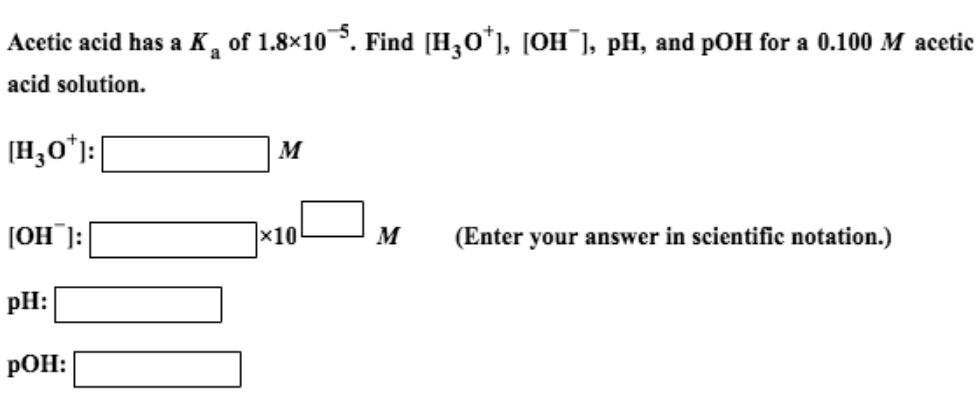# Problem: Acetic acid has a Ka of 1.8 times 10^-5. Find [H3O+], [OH], pH, and pOH for a 0.100 M acetic acid solution. [H3O+]: M [OH]: times 10 M (Enter your answer in scientific notation.)

###### FREE Expert Solution
79% (267 ratings)###### Problem Details

Acetic acid has a Ka of 1.8 times 10^-5. Find [H3O+], [OH], pH, and pOH for a 0.100 M acetic acid solution. [H3O+]: M [OH]: times 10 M (Enter your answer in scientific notation.)